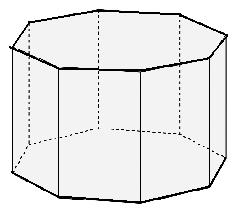Math Calculator

#### Octogonal prism Area, Volume, Surface Area Calculation#### Formula :

Area (A) = 2 × a × d Volume(V) = A × l Surface Area (SA) = (2 × A) + (8 × a × l) Where, a = Side Length l = Height d = Distance